ON INVERSE FUNCTIONS

With Restricted DomainsYou can always find the inverse of a one-to-one function without restricting the domain of the function. Recall that a function is a rule that links an element in the domain to just one number in the range. You could have points (3, 7), (8, 7) and (14,7) on the graph of a function. A one-to-one function adds the requirement that each element in the range is linked to just one number in the domain. In this case, the above three points would not be points on the graph of a one-to-one function because 7 links to different numbers in the domain.

You can identify a one-to-one function from its graph by using the Horizontal Line Test. Mentally scan the graph with a horizontal line; if the line intersects the graph in more than one place, it is not the graph of a one-to-one function. If the function is not one-to-one, then its inverse will not be unique, and the inverse function must be unique. The domain of the original function must be restricted so that its inverse will be unique.

This section will show you how to restrict the domain and then find a unique inverse on that domain. Study the graph of a function that is not one-to-one and choose a part of the graph that is one-to-one. We will find the inverse for just that part of the graph.

The following is an example of finding the inverse of a function that is not one-to-one.

Example 1: Find the inverse of the function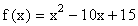.

Solution:

By now you should recognize that this is the equation of a parabola. Since a parabola is "U" shaped, it is not one-to-one. Take a look at the graph below. We know that if we divide the x-axis into two parts at the point x = 5, the graph on the left side of the line x = 5 is one-to-one, and the graph on the right side of the line x = 5 is also one-to-one. The red part of the x-axis is the set of all real numbers in the interval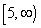, and the blue part of the x-axis is the set of all real numbers in the interval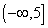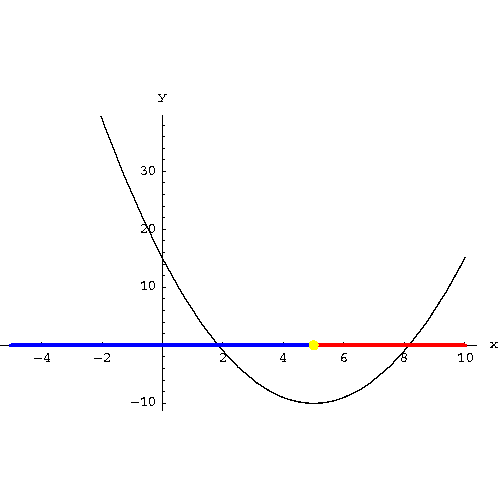You could have also discovered the x = 5 dividing point by finding the vertex of the parabola. You can find the vertex of parabola by rewriting the original equationso that we have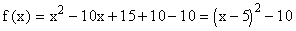. From the last form, we can read the vertex as (5, -10). We are only interested in the x-part of the point, the 5.

• The identify function is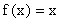. The graph of the identify function is the straight line that bisects the first and third quadrants and passes through the origin. All the points on the graph of this line have an x- and y- coordinate that are equal. (2, 2), (7, 7) et.
• Since an inverse function is a kind of "UNDO" function, the composition of a function with its inverse is the identify function. For example, if the rule f(x) takes a 3 to 10 and the inverse function takes the 10 back to the 3, the end results is that the composite of the two functions took 3 to 3. This is the identify function.
• We know then that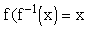. Let’s use the rule for the f(x) function with the argument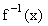and set it equal to x.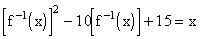• Add 10 to both sides of the above equation.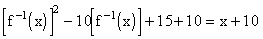• Factor the left side of the equation.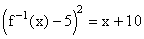• Take the square root of both sides of the equation.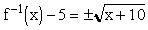• Add 5 to both sides of the equation.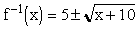• Now we have a problem. We have come up with two inverses and inverses must be unique. Which one shall we choose as the inverse,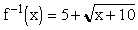or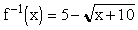? It depends on what you choose as your restricted the domain.
• If you choose the restricted domain to be, the inverse isbecause the range of the inverse is equal to the restricted domain of the original function.
• If you choose the restricted domain to be, the inverse isbecause the range of the inverse is equal to the restricted domain of the original function.

Check:

Suppose you restricted the domain. Choose a number from this domain, say 10. The function f takes the 10 to 15 because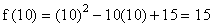. The inverse function takes the 15 to 10 because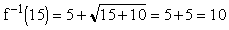Review another example of finding the inverse of a function where the domain of the original function needs to be restricted.

Work the following problems and check the answers and solutions.

2. Find the inverse of the function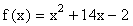. Answer

3. Find the inverse of the function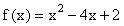. Answer[Back] [Next]
[Algebra] [Trigonometry] [Complex Variables]
[Calculus] [Differential Equations] [Matrix Algebra]S.O.S MATHematics home page

Do you need more help? Please post your question on our S.O.S. Mathematics CyberBoard.Author:Nancy Marcus